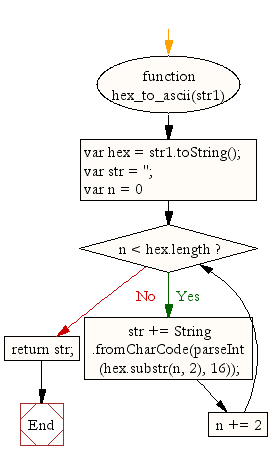# JavaScript: Convert Hexadecimal to ASCII format

## JavaScript String : Exercise-28 with Solution

Write a JavaScript function to convert Hexadecimal to ASCII format.

Test Data:
console.log(hex_to_ascii('3132'));
console.log(hex_to_ascii('313030'));
Output:
"12"
"100"

Sample Solution:-

HTML Code:

``````<!DOCTYPE html>
<html>
<meta charset="utf-8">
<title>Write a JavaScript function to convert Hexadecimal to ASCII</title>
<body>

</body>
</html>
```
```

JavaScript Code:

``````function hex_to_ascii(str1)
{
var hex  = str1.toString();
var str = '';
for (var n = 0; n < hex.length; n += 2) {
str += String.fromCharCode(parseInt(hex.substr(n, 2), 16));
}
return str;
}
console.log(hex_to_ascii('3132'));
console.log(hex_to_ascii('313030'));
```
```

Sample Output:

```12
100
```

Flowchart:Live Demo:

See the Pen JavaScript Convert Hexadecimal to ASCII format - string-ex-28 by w3resource (@w3resource) on CodePen.

Improve this sample solution and post your code through Disqus

What is the difficulty level of this exercise?

﻿

## JavaScript: Tips of the Day

JavaScript: eval function

```const sum = eval('10*10+5');
```

eval evaluates codes that's passed as a string. If it's an expression, like in this case, it evaluates the expression. The expression is 10 * 10 + 5. This returns the number 105.

Ref: https://bit.ly/323Y0P6